# Cross product facts for kids

Kids Encyclopedia Facts

The cross product is a mathematical operation which can be done between two vectors. After performing the Cross Product, a new vector is formed. The cross product of two vectors is always perpendicular to both of the vectors which were "crossed". This means that the cross product must always be used in 3-Dimensional space.

## Importance of the cross product

Being a vector operation, the Cross Product is extremely important in all sorts of sciences (particularly physics), engineering, and mathematics. One important example of the Cross Product is in torque or moment.

## How to perform the cross product

Like any mathematical operation, the Cross Product can be done in a straightforward way.

### Two dimensions

If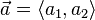$\vec{a} = \langle a_1,a_2 \rangle$
and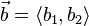$\vec{b} = \langle b_1,b_2 \rangle$
then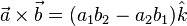$\vec{a} \times \vec{b} = (a_1b_2-a_2b_1)\hat{k}$

or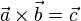$\vec{a} \times \vec{b} = \vec{c}$
and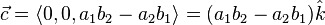$\vec{c} = \langle 0,0,a_1b_2-a_2b_1 \rangle = (a_1b_2-a_2b_1)\hat{k}$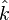$\hat{k}$ is just a symbol which says that our new vector is pointing up (in the z-direction). If you "cross" two vectors which are both in the x-y plane, the product will always be perpendicular to both vectors, and if both vectors are in the x-y plane, the only way for it to be perpendicular to both is to be in the z direction. If the value of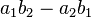$a_1b_2-a_2b_1$ is positive, then it points OUT of the page; if its value is negative, then it points INTO the page.

### Three dimensions

If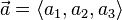$\vec{a} = \langle a_1, a_2, a_3 \rangle$
and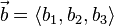$\vec{b} = \langle b_1, b_2, b_3 \rangle$
then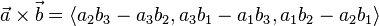$\vec{a} \times \vec{b} = \langle a_2 b_3 - a_3 b_2, a_3 b_1 - a_1 b_3, a_1 b_2 - a_2 b_1 \rangle$

## Basic properties of the cross product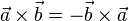$\vec{a} \times \vec{b} = -\vec{b} \times \vec{a}$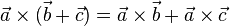$\vec{a} \times (\vec{b} + \vec{c}) = \vec{a} \times \vec{b} + \vec{a} \times \vec{c}$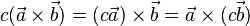$c(\vec{a} \times \vec{b}) = (c\vec{a})\times\vec{b} = \vec{a}\times(c\vec{b})$Cross product Facts for Kids. Kiddle Encyclopedia.